# Planetary motion tackled kinematically

Orbital motion expressed in terms of the auxiliary angle

Introduction

Nowadays astronomers accept that planetary motion has to be treated dynamically, as a many-body problem, for which there is bound to be no exact solution. This situation is currently approximated in textbooks by the two-body problem, which itself became amenable to analysis only after the work of Newton (1642-1727) . His epoch-making publication was the source of recognisably modern formulations of mass, and force, and acceleration (in fact even the concept of resultant - tangential - velocity seems to have been new). It initiated the science of dynamics as we now know it.

Before that breakthrough, planetary motion involved merely a path (a curve), together with a measure of time, represented geometrically: that is, a strictly kinematical treatment. This by definition involves the dimensions of length and time alone, while excluding altogether the dimension of mass. Thus the situation is simplified to one in which a planet (regarded as a point) moves in a plane about a fixed source of motion. (The theory was developed first in terms of circles based on the heliocentric configuration invented by Copernicus (1473-1543) .) In this case, the motion of each individual planet occurs in isolation, entirely unaffected by any other member of the system. This will be specifically referred to as the 'one-body problem'. It is this situation we shall now examine, adjusting our terminology accordingly (in particular, replacing any mention of 'velocity' with the more appropriate term 'motion' throughout). The astronomical solution to the one-body problem consists of the two laws:
• Law I (the Ellipse Law) - the curve or path of a planet is an ellipse whose radius vector is measured from the Sun which is fixed at one focus.

• Law II (the Area Law) - the time taken by a planet to reach a particular position is measured by the area swept out by the radius vector drawn from the fixed Sun.
This composite solution represents what is in fact the earliest instance of a planetary orbit: it will be succinctly referred to in what follows as 'the Sun-focused ellipse'. We shall now prove that, subject to its obvious external limitations, this unique solution is of universal applicability as a self-contained piece of mathematics. Moreover the topic is of great historical significance - since the discovery of the two laws stated above actually took place during the period 1600-1630 , under the kinematical circumstances described above: see Kepler's Planetary Laws. Therefore it is of interest to assess the validity of the techniques actually employed at the time, applying rigorous modern methods as a standard of comparison.

Unexpectedly, this analysis is carried out in terms of the auxiliary angle of the ellipse, rather than the polar angle (at the Sun) that is invariably used nowadays: this came about for historical reasons - because, until the adoption of the heliocentric view, the position of the Sun did not play an explicit part in planetary theory. Moreover, the kinematical solution is qualitatively different from any later, dynamical one in that it possesses exact geometrical representation - while the adoption of the auxiliary angle as variable ensures that the treatment turns out to be the simplest possible.

In what follows, we establish the properties of an ellipse, both as a path, in Part I, and as an orbit, in Part II; while in Part III we will derive Law III, the relationship that synthesizes the planetary system.

Part I. Geometrical properties of the ellipse with focus at the origin

(i) Determination of the radius vectorThe figure shows an ellipse with its major auxiliary circle diameter CD, centre B, whose given measures will be denoted by BC = BD = a, the major semiaxis of the ellipse, and BF = b, its minor semiaxis. The focus A is constructed geometrically by drawing FM parallel to CD to cut the circle at M, and dropping a perpendicular from M to cut CD at A (thus making AM = BF). Then we set AB = BE = ae, where ae is derived from the relationship that connects the three determining constants of an ellipse (it may be referred to as 'the focus-fixing property'):
a2e2 = a2 - b2.             (1)
It is essential to appreciate here that e not only denotes the focal eccentricity (the 'ellipticity') but the polar eccentricity as well, since A is both the focus and the origin or pole of coordinates (which here coincides with the position of the Sun). Otherwise, the present treatment will be ineffective.

By considering the (evidently) congruent right-angled triangles ABF and ABM, we find AF = BM = a. This length AF is subsequently recognized as 'the mean distance', which is of great significance in Part III below.

Our derivation will be carried out exclusively in terms of the auxiliary angle
QBC = β.
This will be unfamiliar to modern readers since the standard treatment is nowadays invariably based on the polar angle PAC = ∠PAC = θ.

We start from what was almost certainly the earliest definition of an ellipse (because it can be derived from the plane section of a cone in three easy steps, as set out in ). It enables the ellipse to be regarded as a 'compressed circle', by a relation known nowadays as 'the ratio-property of the ordinates':
PH/QH = b/a.             (2)
Now from ΔQHB,
QH = asin β,
so from (2),
PH = b/a. QH = b sin β .
We will first find the radius vector AP = r in terms of β (though it will be convenient to introduce the polar angle θ temporarily, in a subsidiary capacity). Then two geometrical equivalences can be derived from ΔAPH, again shown in the figure:
PH = r sin θ = b sin β             (3)

AH = r cos θ = a(cos β + e).             (4)
Applying Pythagoras' theorem to ΔAPH, we derive:
r2 = AP2 = PH2 + AH2
and thus,
r2 = b2sin2 β + a2(cos β + e)2.
Using (1),
r2 =a2(1 - e2)sin2 β + a2(cos2 β + 2e cos β + e2)

= a2(sin2 β - e2sin2 β + cos2 β + 2e cos β + e2)

= a2(1 + 2e cos β + e2cos2β).
Hence,
r = AP = a(1 + e cos β).             (5)
This is Law I: the equation of the elliptic path with respect to the origin at one focus: see Kepler's Planetary Laws: Section 6.

(ii) Proof that the ellipse is 'simpler than any circle (except one)'

We consider the equation of a circle with origin at some eccentric point: as an illustration we may take the circle CQD, centre B, shown in the figure, where A is to be regarded as the origin or pole; just for our present purpose, we set AB = a e to represent the 'polar distance' alone (since the focal distance for a circle is zero). Then we use the information from (i) above to calculate the radius vector AQ of the circle:
AQ2 = AH2 + QH2

= a2(cos β + e)2 + a2sin2 β

= a2(1 + 2e cos β + e2).
So,
AQ = a(1 + 2e cos β + e2)½
Hence,
AQ = a(1 + e cos β + 1/2 e2sin2 β + ...).
Therefore it is clear that this expression for the radius vector of a circle with its origin at an eccentric point is much less simple than that for the radius vector of the ellipse with the same origin when that point is its focus, as set out in (5) just above.

Further, this argument could be generalized by carrying out a similar brief calculation to find the radius vector of any ellipse belonging to the system of conics whose origin is at the Sun (again setting polar distance AB = ae) that has CQD as its auxiliary circle and its typical point lying on QH (still defined by auxiliary angle β). However, because each such ellipse possesses its own individual eccentricity, this would introduce a separate constant (say ε ) to represent the focal eccentricity of that particular ellipse, and thus produce a still more complicated expression. Since both the focal distance and the polar distance are measured from the centre B of the ellipse, it is only when these two distances coincide (aε = ae), uniquely, that we obtain the simplest possible equation -- as expressed in (5). (And mathematicians will not need convincing that the simplest of all circles, having its origin at the centre B, is no more than a special case of that system of conics, with e = ε = 0.)

(iii) Evaluation of the transradial arc r dθ

From the equivalences for PH set out in (3), we obtain:
sinθ/sin β = b/r.            (6)
So, applying the formula for the radius vector from (5), we have:
sin θ = b/a sinβ/1 + e cos β .
Differentiating with respect to β, we derive: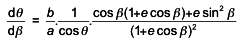and using (4),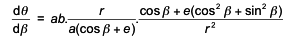.
Hence,
dθ/dβ = b/r.            (7)
This identity acts as the bridging relation (inverse or direct) between the modern treatment by polar angle and the present treatment by auxiliary angle (which will only work effectively in the case of the unique Sun-focused ellipse alone).

Moreover, this purely geometrical relationship is unexpectedly of enormous significance in connection with one kinematical component of the orbit, as we shall see in Part II(i) below. Meanwhile we point out that the transradial arc is constant with respect to the auxiliary angle:
r dθ = b dβ.            (8)

Part II. Kinematical properties of the ellipse with focus at the origin

(i) The transradial component of motion rdθ/dt
(This motion is known to some mathematicians as the transverse component of motion). Whatever we call it, this motion is defined to take place round the Sun instantaneously in a circle.

The characteristic property of orbital motion in its most general form is generally stated dynamically, but it was in fact first proved as a kinematical relation in Book I, Prop.1 of Newton's work, already cited  (at that early stage, the concept of mass had not yet been introduced). This property can be formulated in various ways, all equivalent to the statement that equal areas correspond to equal times. The constant of proportionality involved (1/2h is standard usage) is expressed mathematically by the following relationship, in which r represents the radius vector measured from the source of motion at the Sun, still taken as the origin of coordinates, again with reference to the figure:
r2 dθ/dt = h.
This is the modern mathematical expression of the kinematical area-time law.

We now apply this to the special case of the Sun-focused ellipse, whose total area is π ab and periodic time T, in order to evaluate its particular constant. For one complete circuit, the area-time law gives:
ab/T = h.            (9)
So in this case,
r2 dθ/dt = 2π ab/T,
and hence,
r dθ/dt = 2π ab/T × 1/r .            (10)
Now from the evaluation of the transradial arc in (8) above, we have:
r dθ/dt = b dβ/dt .            (11)
Thus for the Sun-focused ellipse alone, we deduce from (10) and (11):
dβ/dt = 2π a/T × 1/r.            (12)
We digress to consider the inverse form:
dt/dβ = T/ × r/a = T/ (1 + e cos β)    from (5).
Hence by integration,
tβ + e sin β.
This is Law II: the time expressed in angular measure. Then, by introducing the dimensional constant 1/2 ab, for the Sun-focused ellipse alone, we can easily deduce that time is proportional to area. See Kepler's Planetary Laws: Section 7.

[For a less precise version of equation (10) - simply that the transradial motion is proportional (inverse-linearly) to the distance - see Kepler's Planetary Laws: Section 10.]

(ii) The radial component of motion dr/dt
This motion takes place linearly in the direction of the radius vector -- towards or away from the Sun.

r = a(1 + e cos β).
Hence,
dr/dβ = -ae sin β.            (13)
This is the radial variation of the distance with respect to β: see Kepler's Planetary Laws: Section 11.]

Continuing our modern treatment, we carry out a change of variable, using (12) and (13):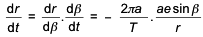,
and thus,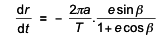.            (14)
It can easily be checked that calculating the resultant of these two components (10) and (14) will produce the modern value of the 'velocity' in orbit -- but there is no reason to do so since the present treatment by components is entirely adequate -- and much simpler -- for a kinematical approach.

On the other hand, for the removal of doubt, we should confirm that this treatment is compatible with the modern dynamical approach, by determining the acceleration that corresponds to this motion (as has been said, this concept was an anachronism in Kepler's day). There are several ways of carrying this out, which unfortunately involve either sophisticated calculus or fairly heavy algebra. We start from the formula analogous to that found in textbooks of dynamics:
Radial acceleration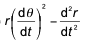directed towards the Sun.            (15)
Then we apply result (11) above to the first term, and, as one possibility for the second term, introduce a formula for change of variable which is found in some calculus textbooks: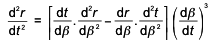.            (16)
This can be expressed in terms of r as required by differentiating (12) and (13), and also using (14), and then simplified by applying (5) and (1). Lastly by using (12), we obtain:
Radial acceleration = (2π)2 a3/T2 × 1/r2 towards the Sun.
Now introducing, provisionally, the quantity μ0 to represent (2π)2 a3/T2, we express the radial acceleration in the more familiar form:
Acceleration = μ0/r2 towards the Sun.
This quantity μ0 is evidently determined by the particular orbit, and thus appears to be a (kinematical) constant associated with the individual planet. It will be interpreted further in Part III below.

We conclude that this theory is rigorously exact in kinematical terms for an individual planet, in accordance with presentday standards. Moreover, subject to precise determination of the values of all the constants involved, Kepler's own treatment was entirely satisfactory, up to the level of first order differentiation.

Part III. Corollary: the derivation of Law III for a system of planets

A geometrical lemma to Part I(i) above will enable us to evaluate AL = l, the semilatus rectum, shown in the figure (where L is the point of the ellipse lying on AM). By the original construction, AM = BF = b. Accordingly, applying the ratio-property of the ordinates, we obtain:
AL/AM = l/b = b/a.
Hence,
b2 = al.            (17)
Now we return to equation (9), which stated the area-time law (in kinematical terms) for one complete circuit:
h = 2π ab/T,
and so,
h2 = (2π)2a2b2/T2
Using (17), we obtain:
h2 = (2π)2a3l/T2.
Rearranging,
a3/T2 = 1/(2π)2.h2/l .
Since h and l are constants determined by the particular orbit, we will follow Cohen  (who presumably chose the notation to commemorate the discoverer of this relationship) to write the result:
a3/T2 = K.            (18)
Hence we have uncovered the existence of a kinematical relationship between the square of the periodic time and the cube of the mean distance for each of the (six) planets independently, each apparently possessing its own individual value of K. In the event the value of K was compared empirically for all the planets in pairs, and was found to be constant (within observational limits) for every pair tested; K was then assumed to have a common value for the whole planetary system -and the relationship is known as Kepler's Third Law . (It was proved, in a dynamical context, in Book I, Prop.15 of Newton's work, already cited.) However, it is possible to formulate a rational basis for the above deduction, founded on geometry - and so to produce a theoretical proof of Law III which would have been not so far beyond the conceptual understanding of a pre-Newtonian mathematician . (The proof involves the extension of a method actually used by Kepler in his proof of the area law.)

Accordingly, it is evident that the quantity μ0 provisionally defined in Part II(iii) - there associated with an individual planet -- may now be identified as a kinematical constant that will operate to synthesize the planetary system. (It is explained in elementary textbooks of modern astronomy that the corresponding value μ in the dynamical system depends on the relative masses as well as the actual constant of gravitation.) So we will name μ0 'the coefficient of planetary cohesion', and in correlation, we have:
μ0 = (2π)2 .a3/T2 = (2π)2K.

Article by: A E L Davis, University College, London.

List of References (7 books/articles)

 JOC/EFR © October 2006 Copyright information School of Mathematics and Statistics University of St Andrews, Scotland